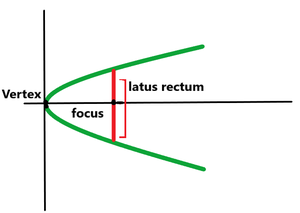# Program to find the length of Latus Rectum of a Parabola

• Last Updated : 28 Jul, 2021

Given a, b, and c the coefficients of X2, X, and the constant term in the general equation of the parabola, the task is to find the length of the Latus Rectum of the parabola.

Examples:

Attention reader! Don’t stop learning now. Get hold of all the important DSA concepts with the DSA Self Paced Course at a student-friendly price and become industry ready.  To complete your preparation from learning a language to DS Algo and many more,  please refer Complete Interview Preparation Course.

In case you wish to attend live classes with experts, please refer DSA Live Classes for Working Professionals and Competitive Programming Live for Students.

Input: a = 3, b = 5, c = 1
Output: 0.333333

Input: a = 4, b = 0, c = 4
Output: 0.25

Approach: The given problem can be solved based on the following observations:

Observation:

• The Latus rectum of a parabola is the perpendicular line to the axis and at the focus of parabola and its length is equal to 4 times the distance between the focus and vertex of the parabola.• Therefore, the task is reduced to find the distance between focus and vertex of the parabola using formula:
•Follow the steps below to solve the problem:

• Initialize two variables, say vertex and focus to store the coordinates of vertex and focus of the parabola.
• Find the coordinates of the vertex and focus of the parabola and store in corresponding variables.
• Initialize a variable, say length, and set it to 4 times the distance between vertex and focus of the parabola.
• Print the value of length as the answer.

Below is the implementation of the above approach:

## C++

 // C++ program for the above approach #include using namespace std; // Function to calculate distance// between two pointsfloat distance(float x1, float y1,               float x2, float y2){    // Calculating distance    return sqrt((x2 - x1) * (x2 - x1)                + (y2 - y1) * (y2 - y1));} // Function to calculate length of// the latus rectum of a parabolafloat lengthOfLatusRectum(float a,                          float b, float c){    // Stores the co-ordinates of    // the vertex of the parabola    pair<float, float> vertex        = { (-b / (2 * a)),            (((4 * a * c) - (b * b)) / (4 * a)) };     // Stores the co-ordinates of    // the focus of parabola    pair<float, float> focus        = { (-b / (2 * a)),            (((4 * a * c) - (b * b) + 1) / (4 * a)) };     // Print the distance between focus and vertex    cout << 4 * distance(                    focus.first, focus.second,                    vertex.first, vertex.second);} // Driver Codeint main(){    // Given a, b & c    float a = 3, b = 5, c = 1;     // Function call    lengthOfLatusRectum(a, b, c);     return 0;}

## Java

 // Java program for the above approachclass GFG{  static class pair  {    float first;    float second;    public pair(float first, float second)     {      this.first = first;      this.second = second;    }     }   // Function to calculate distance  // between two points  static float distance(float x1, float y1,                        float x2, float y2)  {     // Calculating distance    return (float) Math.sqrt((x2 - x1) * (x2 - x1)                             + (y2 - y1) * (y2 - y1));  }   // Function to calculate length of  // the latus rectum of a parabola  static void lengthOfLatusRectum(float a,                                  float b, float c)  {     // Stores the co-ordinates of    // the vertex of the parabola    pair vertex      = new pair( (-b / (2 * a)),                 (((4 * a * c) - (b * b)) / (4 * a)) );     // Stores the co-ordinates of    // the focus of parabola    pair focus      = new pair( (-b / (2 * a)),                 (((4 * a * c) - (b * b) + 1) / (4 * a)) );     // Print the distance between focus and vertex    System.out.print(4 * distance(      (float)focus.first, (float)focus.second,      (float)vertex.first, (float)vertex.second));  }   // Driver Code  public static void main(String[] args)  {     // Given a, b & c    float a = 3, b = 5, c = 1;     // Function call    lengthOfLatusRectum(a, b, c);   }} // This code is contributed by 29AjayKumar

## Python3

 # Python 3 program for the above approachfrom math import sqrt # Function to calculate distance# between two pointsdef distance(x1, y1, x2, y2):       # Calculating distance    return sqrt((x2 - x1) * (x2 - x1) + (y2 - y1) * (y2 - y1)) # Function to calculate length of# the latus rectum of a paraboladef lengthOfLatusRectum(a, b, c):       # Stores the co-ordinates of    # the vertex of the parabola    vertex =  [(-b / (2 * a)), (((4 * a * c) - (b * b)) / (4 * a))]     # Stores the co-ordinates of    # the focus of parabola    focus = [(-b / (2 * a)), (((4 * a * c) - (b * b) + 1) / (4 * a))]     # Print the distance between focus and vertex    print("{:.6f}".format(4 * distance(focus, focus, vertex, vertex))) # Driver Codeif __name__  == "__main__":       # Given a, b & c    a = 3    b = 5    c = 1     # Function call    lengthOfLatusRectum(a, b, c)         # This code is contributed by bgangwar59.

## C#

 // C# program for the above approachusing System; public class GFG{  class pair  {    public float first;    public float second;    public pair(float first, float second)     {      this.first = first;      this.second = second;    }     }   // Function to calculate distance  // between two points  static float distance(float x1, float y1,                        float x2, float y2)  {     // Calculating distance    return (float) Math.Sqrt((x2 - x1) * (x2 - x1)                             + (y2 - y1) * (y2 - y1));  }   // Function to calculate length of  // the latus rectum of a parabola  static void lengthOfLatusRectum(float a,                                  float b, float c)  {     // Stores the co-ordinates of    // the vertex of the parabola    pair vertex      = new pair( (-b / (2 * a)),                 (((4 * a * c) - (b * b)) / (4 * a)) );     // Stores the co-ordinates of    // the focus of parabola    pair focus      = new pair( (-b / (2 * a)),                 (((4 * a * c) - (b * b) + 1) / (4 * a)) );     // Print the distance between focus and vertex    Console.Write(4 * distance(      (float)focus.first, (float)focus.second,      (float)vertex.first, (float)vertex.second));  }   // Driver Code  public static void Main(String[] args)  {     // Given a, b & c    float a = 3, b = 5, c = 1;     // Function call    lengthOfLatusRectum(a, b, c);   }}    // This code is contributed by 29AjayKumar

## Javascript

 

Output:
0.333333

Time Complexity: O(1)
Auxiliary Space: O(1)

My Personal Notes arrow_drop_up